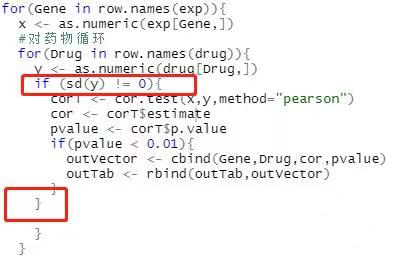Posted on

CellMiner数据库，主要是通过国家癌症研究所癌症研究中心(NCI)所列出的60种癌细胞为基础而建立的。该数据库最初发表于2009年，后于2012年在Cancer Research杂志上进行了更新，题目为“CellMiner: a web-based suite of genomic and pharmacologic tools to explore transcript and drug patterns in the NCI-60 cell line set”。大家后期在使用该数据库记得应用相关文献。

NCI-60细胞系是目前使用最广泛的用于抗癌药物测试的癌细胞样本群。大家可以通过它查询到 NCI-60细胞系中已确认的22379个基因，以及20503个已分析的化合物的数据（包括多种已获美国食品和药物监督局批准的药物，以及临床试验中的药物分子）。

1.CellMiner数据库的使用

1. 进入CellMiner数据库主页https://discover.nci.nih.gov/cellminer/home.do）；

3. 在此，我们直接选择经过处理后的数据Processed Data Set，勾选RNA表达数据（RNA: RNA-seq）和药物数据（Compound activity: DTP NCI-60）；

4. 点击按钮Get Processed Set，进入下载界面，点击即可保存；

5.下载完成后，将其放到工作目录下，解压，并分别提取其中的Excel文件放于当前工作目录下；

2.药物数据的准备

2.1 读取药物相关数据

``````library(readxl)
rt1 <- read_excel(path = "DTP_NCI60_ZSCORE.xls", skip = 7)
``````

``````colnames(rt1) <- rt1[1,]
rt1 <- rt1[-1,-c(67,68)]
``````

2.2 筛选药物标准

``table(rt1\$`FDA status`)``

``````rt1 <- rt1[rt1\$`FDA status` %in% c("FDA approved", "Clinical trial"),]
rt1 <- rt1[,-c(1, 3:6)]
write.table(rt1, file = "drug.txt",sep = "\t",row.names = F,quote = F)
``````

3. 基因表达数据的准备

``````rt2 <- read_excel(path = "RNA__RNA_seq_composite_expression.xls", skip = 9)
colnames(rt2) <- rt2[1,]rt2 <- rt2[-1,-c(2:6)]
write.table(rt2, file = "geneExp.txt",sep = "\t",row.names = F,quote = F)
``````

4. 药物敏感性分析

``rm(list = ls())``

4.1 引用包

``````library(impute)
library(limma)
``````

4.2 读取药物输入文件

``````rt <- read.table("drug.txt",sep="\t",header=T,check.names=F)
rt <- as.matrix(rt)rownames(rt) <- rt[,1]
drug <- rt[,2:ncol(rt)]
dimnames <- list(rownames(drug),colnames(drug))
data <- matrix(as.numeric(as.matrix(drug)),nrow=nrow(drug),dimnames=dimnames)
``````

``````mat <- impute.knn(data)
drug <- mat\$data
drug <- avereps(drug)
``````

4.3 读取表达输入文件

``````exp <- read.table("geneExp.txt", sep="\t", header=T, row.names = 1, check.names=F)
dim(exp)exp[1:4, 1:4]
``````

4.4 提取特定基因表达

``````gene <- read.table("gene.txt",sep="\t",header=F,check.names=F)
genelist <- as.vector(gene[,1])
genelist
``````

``````genelist <- gsub(" ","",genelist)
genelist <- intersect(genelist,row.names(exp))
exp <- exp[genelist,]
``````

4.5 药物敏感性计算

``````outTab <- data.frame()
for(Gene in row.names(exp)){
x <- as.numeric(exp[Gene,])
#对药物循环
for(Drug in row.names(drug)){
y <- as.numeric(drug[Drug,])
corT <- cor.test(x,y,method="pearson")
cor <- corT\$estimate
pvalue <- corT\$p.value
if(pvalue < 0.01){
outVector <- cbind(Gene,Drug,cor,pvalue)
outTab <- rbind(outTab,outVector)
}
}
``````

``````outTab <- outTab[order(as.numeric(as.vector(outTab\$pvalue))),]
write.table(outTab, file="drugCor.txt", sep="\t", row.names=F, quote=F)
``````

## 31 Replies to “cellMiner数据库的使用及药物敏感性预测”

1.古小月说道：

进哥你好，打扰请教一下：
我在运行这个代码的第四部分的时候， 采用for循环进行药物敏感性计算，一直报错：Error in if (pvalue < 0.01) { : missing value where TRUE/FALSE needed，后反复检查，发现对drug矩阵进行row.names(drug)操作的时候，就会陷入巨大的计算量，一直进入卡死状态，后来我发现只要对这个drug矩阵的行名进行任何操作都会进入卡死状态，如果把行名变成第一列之后，对第一列进行读取还是一样的结果，各种检查都不知道为什么，请问进哥是否遇到过这类问题？
麻烦啦。

1.进哥哥说道：

你好 如果还没解决，方便的话加我微信，我看一下数据

2.Lynn说道：

你好，我按照上诉代码跑结果，最后一步时出现了问题，请问王老师能否抽空回复一下
最后一步画barplot组合图时，p值全是ns，反馈结果如下：
> ggarrange(plotlist=plotList_2,nrow=nrow,ncol=ncol)
Warning messages:
1: In wilcox.test.default(c(-1.61, -1.49, -1.54, 0.19, 0.9, 0.95, -0.29, :
无法精確計算带连结的p值
2: In wilcox.test.default(c(-2.81, -1.14, -0.67, -0.57, -0.5, 0, -2, :
无法精確計算带连结的p值
3: In wilcox.test.default(c(-1.61, -1.61, -1.13, -0.29, 0.06, -1.37, :
无法精確計算带连结的p值
4: In wilcox.test.default(c(-0.31, -1.09, -0.44, -0.06, 0.06, 0.11, :
无法精確計算带连结的p值
5: In wilcox.test.default(c(-0.44, -0.55, -1.44, 0.5, 0.1, 0.42, -1.93, :
无法精確計算带连结的p值
6: In wilcox.test.default(c(-1.66, -1.77, 0.96, -0.39, -0.42, -0.54, :
无法精確計算带连结的p值
7: In wilcox.test.default(c(-0.8, -0.8, -0.7, -0.8, -0.18, -0.75, -0.8, :
无法精確計算带连结的p值
8: In wilcox.test.default(c(-1.15, -1.29, -0.73, -0.64, 0.29, -0.59, :
无法精確計算带连结的p值
9: In wilcox.test.default(c(-1.2, -1.39, -0.27, -0.69, 0.22, 0.66, :
无法精確計算带连结的p值

1.Lynn说道：

不好意思老师，才发现后面的代码是别处复制过来的
plotList_2 <- list()
corPlotNum <- 16
if(nrow(outTab)<corPlotNum){
corPlotNum=nrow(outTab)
}

for(i in 1:corPlotNum){
Gene <- outTab[i,1]
Drug <- outTab[i,2]
x <- as.numeric(exp[Gene,])
y <- as.numeric(drug[Drug,])
df1 <- as.data.frame(cbind(x,y))
colnames(df1) <- "IC50"
df1\$group median(df1\$x), “high”, “low”)
compaired <- list(c("low", "high"))
p1 <- ggboxplot(df1,
x = "group", y = "IC50",
fill = "group", palette = c("#00AFBB", "#E7B800"),
add = "jitter", size = 0.5,
xlab = paste0("The expression of ", Gene),
ylab = paste0("IC50 of ", Drug)) +
stat_compare_means(comparisons = compaired,
method = "wilcox.test", #设置统计方法
symnum.args=list(cutpoints = c(0, 0.001, 0.01, 0.05, 1),
symbols = c("***", "**", "*", "ns")))
plotList_2[[i]]=p1
}

nrow <- ceiling(sqrt(corPlotNum))
ncol <- ceiling(corPlotNum/nrow)
ggarrange(plotlist=plotList_1,nrow=nrow,ncol=ncol)
ggarrange(plotlist=plotList_2,nrow=nrow,ncol=ncol)

1.进哥哥说道：

直接加我微信吧 微信交流 需要你绘图的文件 这样我方便修改

3.zhou说道：

您好，我用的TCGA数据库通过分析将患者分成高低风险两组，对比两组的药敏分析，我要如何准备数据呢

1.进哥哥说道：

您好，就是分别计算两组的风险评分与药物敏感性的相关性，药物相关数据参考文中的 你的数据就是高低风险组各个样本的风险评分

1.果果王说道：

想问问具体代码可以指点下嘛，用的TCGA数据库通过分析将患者分成高低风险两组，对比两组的药敏分析

1.进哥哥说道：

我先解释一下计算过程 不懂加微信讨论
你应该已经由你的训练数据得到一个计算Risk score的signatures和计算公式 将此公式应用于cellminer中基因表达数据集，由此得到各种细胞的risk score 再计算这个score与药物敏感性数据的相关性

1.说道：

您好，请问方便加您微信吗？我也是分组后，关于比较两组之间药物敏感性的问题想请教下您

2.进哥哥说道：

不好意思 前两天忙备课高校教资考试，可以的 导航栏我的简历有手机号和微信

4.设定新手说道：

运行：
for(Gene in row.names(exp)){
x <- as.numeric(exp[Gene,])
#对药物循环
for(Drug in row.names(drug)){
y <- as.numeric(drug[Drug,])
corT <- cor.test(x,y,method="pearson")
cor <- corT\$estimate
pvalue <- corT\$p.value
if(pvalue < 0.05){
outVector <- cbind(Gene,Drug,cor,pvalue)
outTab <- rbind(outTab,outVector)
}
}
}
时出现：
Error in if (pvalue < 0.05) { : missing value where TRUE/FALSE needed
In cor(x, y) : 标准差为零，
这个是怎么回事呢

1.进哥哥说道：

你好，如报错信息，标准差为0，不能做相关性分析，因为有些列数据都一样，导致sd=0，
可以加一个if判断
不清楚加我微信交流

1.设定新手说道：

太感谢了，跑通了

1.panhj说道：

同问怎么加if判断呢，我也是报了同样的错

1.进哥哥说道：

请参考如下修改：2.玊尔说道：

具体怎么加if判断呢，我也是报了同样的错

1.进哥哥说道：

请参考如下修改：

1.panhj说道：

具体怎么加if判断呢，我也是报了同样的错

2.进哥哥说道：

不好意思 之前链接没有加上，请参考如下修改：5.同啊同说道：

你好，Error in impute.knn(data) : a column has more than 80 % missing values!
这个错误怎么解决呢?补齐数据补不上，呜呜

1.进哥哥说道：

你好，正如报错提示，因为缺失值太多，knn法不能准确地补充缺失值，这个时候你需要先把缺失值过多的基因删除，比如我会把缺失值多于60%的基因删除
可以用Na_ratio <- apply(data,2,function(x) sum(is.na(x))/length(x))>0.6计算得到各列确实值比例，然后data[-Na_ratio]即可

1.叶子说道：

大佬好，以下是我练习的流程，小白一个
#开始药物敏感性分析
rm(list = ls())
library(impute)
library(limma)

#读取药物输入文件 读取前面保存的药物敏感性结果，设置相应的行名，并将其转换为矩阵形式。
#因为报错和有些数据缺失，我加了个quote="" 不知问题大不大？
rt <- as.matrix(rt)
rownames(rt) <- rt[,1]
drug <- rt[,2:ncol(rt)]
dimnames <- list(rownames(drug),colnames(drug))

data <- matrix(as.numeric(as.matrix(drug)),nrow=nrow(drug),dimnames=dimnames)
#这里也因为转换的原因报错了NAs introduced by coercion
#这样加可以吗？
Na_ratio 0.6
data[-Na_ratio]

#通过impute.knn()函数来评估并补齐药物数据
mat 0.6的一列后，还是出现同样的报错
初学者，问题可能很蠢，求大佬解答

1.叶子说道：

data <- matrix(as.numeric(as.matrix(drug)),nrow=nrow(drug),dimnames=dimnames)
Na_ratio 0.6
data[-Na_ratio]
更正一下

1.叶子说道：

Na_ratio 0.6 出不来

2.进哥哥说道：

不好意思 前两天太忙 没时间回复网站留言，可以的话你直接加微信交流吧

6.陈静说道：

你好，我按照上诉代码不能完全跑出上述结果，出现的问题：感觉R代码不全，不知能否帮忙指导：
在第4.5 药物敏感性计算时，运行
outTab <- data.frame()
for(Gene in row.names(exp)){
x <- as.numeric(exp[Gene,])
#对药物循环
for(Drug in row.names(drug)){
y <- as.numeric(drug[Drug,])
corT <- cor.test(x,y,method="pearson")
cor <- corT\$estimate
pvalue <- corT\$p.value
if(pvalue < 0.01){
outVector <- cbind(Gene,Drug,cor,pvalue)
outTab <- rbind(outTab,outVector)
}
}

出现该错误：
Error in rbind(outTab, outVector) :
cannot coerce type 'closure' to vector of type 'list'

1.进哥哥说道：

您好，看一下中断时的位置，也就是drug是哪个？单独运行查看原因，推测是由于某个药物数据较多缺失值，搞不定加我微信远程看看

7.Jolie说道：

您好，想请教一个问题：cellminer中做出来的药物敏感性拟合图，为什么纵坐标IC50，但基因表达与ic50正相关时代表基因对此药物敏感度高？ IC50 不是越低药物才越有效，细胞才越敏感么？

1.进哥哥说道：

您好，您为什么说纵坐标是IC50，它的单位在数据库中是个Z score，反映的就是药物敏感性指数

1.Jolie说道：

感谢您的回复。我是刚开始看生信文章，有篇文章作者的Supplementary Figure 3 纵坐标是ic50，给了我误解…doi: 10.3389/fonc.2022.830174, 再次感谢您的回复